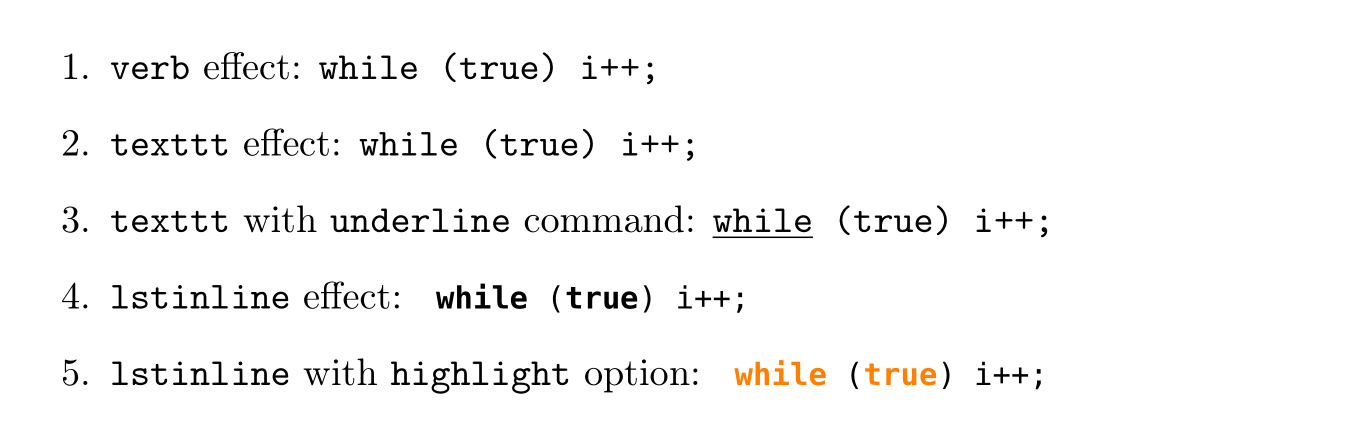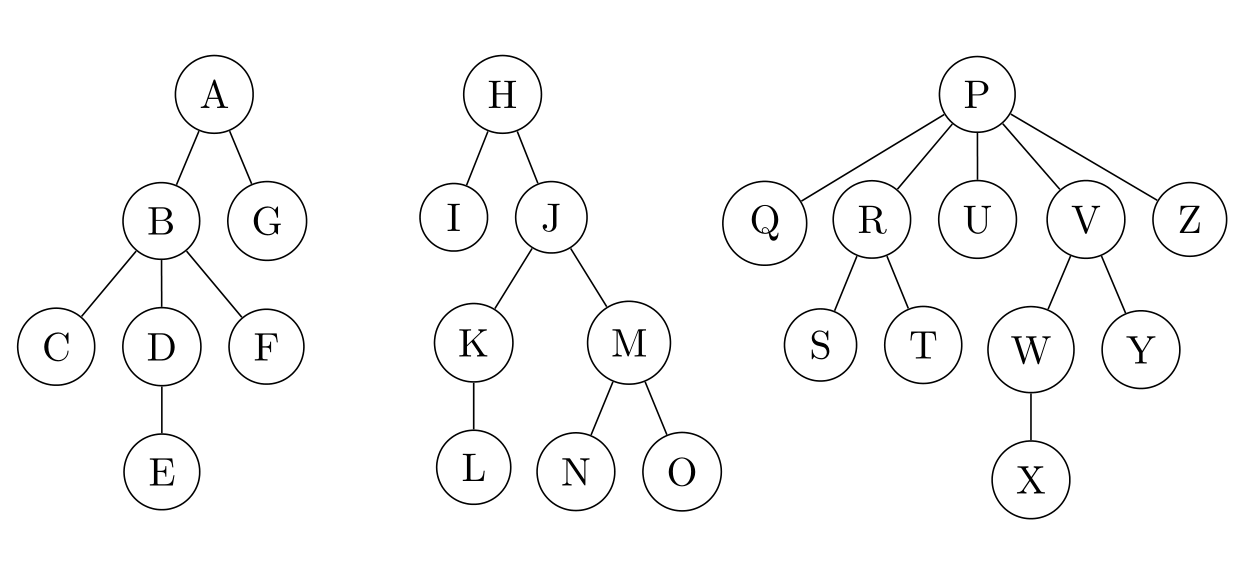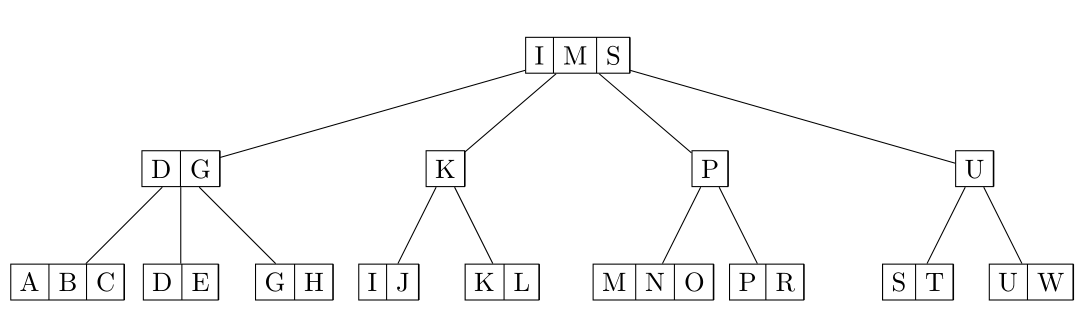### 基础内容

##### 封面
\documentclass[titlepage]{article}

\title{
Homework 1 (Chapter 3) - Due 17th Sep, 2017\\
\begin{large}
Data Structures and Algorithm Analysis in C++
\end{large}
}
\author{Kingsley}
\date{\today}

\begin{document}
\maketitle
blabla...
\end{document}


titlepage 选项 和 \maketitle 命令一起，作用是把标题部分垂直居中作为单独的一页，当然内容比较少的话也可以不让它作为封面。实际交上去的版本，因为是作业，建议把 \author{} 的值换成名字的拼音然后加个空格写上学号。

##### 目录

\clearpage
\tableofcontents
\clearpage


\subsection*{Not Numbered Subsection}


\addcontentsline{file}{sec_unit}{entry}


##### 缩进

LaTeX 默认对 \section{}\subsection{} 下的首段不加缩进，而对于非首段的段落默认都是有缩进的。那如果因为一些原因需要让首段有缩进的话，可以使用 indentfirst 包，只要在文件头部引入这个包就可以了，不需要其他的设置命令：

\usepackage{indentfirst}


##### 字母编号列表

LaTeX 默认的编号列表是数字的，要使用字母编号就需要通过 enumitem 包来指定 label 的格式：

% \usepackage{enumitem}

\begin{enumerate}[label=(\alph*)]
\item $\Theta(n)$
\end{enumerate}


##### 图片

\begin{center}
\includegraphics[width=1\textwidth]{figures/all-probing.jpg}
\end{center}


### 代码

##### 行内代码

• \verb|inline code|
这个命令的作用是直接把两条竖线中间的内容作为行内代码显示出来，如果代码里面包含了竖线的话，为了避免不必要的麻烦可以把起止符号换成感叹号 !

• \texttt{inline code}
这个命令实际上是将内部的文字设置为打印机字体，效果上也可以作为行内代码。此外，它还可以嵌套其他 LaTeX 命令，比如对内部某个词加下划线等：

\texttt{\underline{while} (true) i++;}

• \lstinline{inline code}
这个是 listings 包里的 \lstinline 命令，本身就是用于排版代码的。它还可以和别的包（比如 xcolor）配合，高亮部分保留字：
% \usepackage{listings}
% \usepackage{xcolor}

\lstset{language=C,keywordstyle={\bfseries \color{orange}}}
\lstinline{while (true) i++;}

##### 区块代码

% \usepackage[T1]{fontenc}

\lstset{
basicstyle=
\def\fvm@Scale{0.8}
\fontfamily{fvm}\selectfont,
tabsize=4
}


% \usepackage{listings}

\begin{lstlisting}
#include <iostream>

int main()
{
std::cout << "Hello, World!\n";
return 0;
}
\end{lstlisting}


##### 文件代码

% \usepackage{listings}

\lstinputlisting{sample.cpp}


\lstinputlisting[firstline=3, lastline=7]{sample.cpp}

##### 样式设置

% \usepackage{listings}

\lstdefinestyle{sample-style}{
xleftmargin=2\parindent
}

\lstinline[style=sample-style]{while (true) i++;}
\lstinputlisting[style=sample-style]{sample.cpp}
\begin{lstlisting}[style=sample-style]
// code block
\end{lstlisting}


##### 效果图### 树

##### 二叉树

% \usepackage{tikz}

\begin{tikzpicture}[
every node/.style={
circle, draw,
inner sep=0pt,
text width=6mm,
align=center
},
level distance=10mm,
level 1/.style={sibling distance=30mm},
level 2/.style={sibling distance=15mm}
]
\node{$15$}
child { node{$5$}
child[missing]
child { node{$7$} }
}
child { node{$20$}
child { node{$18$}
child { node{$16$} }
child[missing]
}
child { node{$25$} }
};
\end{tikzpicture}


##### 普通树
###### 使用 tikz 包

% \usepackage{tikz}

\node{X}
child { node{P}
child { node{C} }
child { node{Q} }
child { node{R}
child { node{V} }
child { node{M} }
}
};

###### 使用 forest 包

% \usepackage{forest}

\begin{forest}
[, phantom, s sep = 1cm
[1
[2]
[4]

]
]
\end{forest}


##### 森林

% \usepackage{forest}

\begin{forest}
[, phantom, s sep = 1cm
[A
[B [C] [D[E]] [F]]
[G]]
[H
[I]
[J [K [L]]
[M [N] [O]]]]
[P
[Q] [R [S] [T]] [U] [V [W [X]] [Y]] [Z]]
]
\end{forest}


% \usepackage{forest}

\forestset{
forest-style/.style={
for tree={
circle, draw,
every node/.style={
circle, draw,
inner sep=0pt,
text width=6mm,
align=center
}
}
}
}

\begin{forest} forest-style
[, phantom, s sep = 1cm
// Forest Content
\end{forest}### B+ 树

% \usepackage{tikz}

\usetikzlibrary{shapes}

\begin{tikzpicture}
\tikzstyle{bplus-node}=
[
draw, rectangle split,
rectangle split horizontal,
rectangle split ignore empty parts
]
\tikzstyle{every node}=[bplus-node]

\tikzstyle{level 1}=[sibling distance=35mm]
\tikzstyle{level 2}=[sibling distance=15mm]

\node {I \nodepart{two} M \nodepart{three} S} [-]
child {node {D \nodepart{two} G}
child {node {A \nodepart{two} B \nodepart{three} C}}
child {node {D \nodepart{two} E}}
child {node {G \nodepart{two} H}}
}
child {node {K}
child {node {I \nodepart{two} J}}
child {node {K \nodepart{two} L}}
}
child {node {P}
child {node {M \nodepart{two} N \nodepart{three} O}}
child {node {P \nodepart{two} R}}
}
child {node {U}
child {node {S \nodepart{two} T}}
child {node {U \nodepart{two} W}}
}
;
\end{tikzpicture}### 延伸参考

1. CTAN
这里收录了大部分的 TeX 包以及他们的 README、官方文档等内容，非常详尽。在我感叹 tikz 这个包怎么功能这么强大的时候，我找到了这个包的 文档，然后就……emmm

2. Share LaTeX
这个网站本身是用于在线合作编写 LaTeX 文档的，他们提供的这个 learn 部分感觉对于学习 LaTeX 很有帮助。我记得我高中的时候也有了解过这个网站，因为当时它给的 Demo 里有一张青蛙的图片，让我印象很深刻（

3. WikiBook
搜索结果里经常也看到来自这里的内容，不过我没太仔细了解，应该和上面的那个差不多。相对官方文档而言，这种整合并且带索引的结果可能有时候更符合我们的需求。